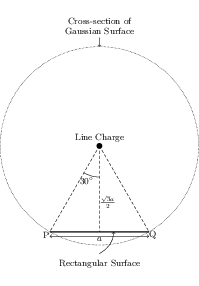# Electric Field due to Infinitely Long Straight Wire

## Problems from IIT JEE

Problem (IIT JEE 2015):An infinitely long uniform charge distribution of charge per unit length $\lambda$ lies parallel to the $y$-axis in the $y\text{-}z$ plane at $z=\frac{\sqrt{3}}{2}a$ (see figure). If the magnitude of the flux of the electric field through the rectangular surface ABCD lying in the $x\text{-}y$ plane with its centre at the origin is $\lambda L/(n\epsilon_0)$ ($\epsilon_0=$ permitivity of free space), then the value of $n$ is,

Solution:The situation is shown in the figure. Consider a cylindrical Gaussian surface of radius $a\sqrt{3}/2$ and length $L$. The charge enclosed by the Gaussian surface is $q_\text{enc}=\lambda L$. Apply Gauss's law to get total flux through the Gaussian surface, \begin{align} \phi={q_\text{enc}}/{\epsilon_0}=\lambda L/\epsilon_0.\nonumber \end{align} The flux through the rectangular surface is equal to the flux through the cylindrical section with chord PQ (see figure). From geometry, the chord PQ subtends an angle $\alpha={60}\;\mathrm{deg}$ at the axis of the cylinder. Thus, the flux through the rectangular surface is, \begin{align} \phi_\text{rectangle}=\phi\,(\alpha/360)=(\lambda L/\epsilon_0)\,(60/360)=\lambda L/(6\epsilon_0).\nonumber \end{align}